# Random bits, true and unbiased, from atmospheric turbulence

## Abstract

Random numbers represent a fundamental ingredient for secure communications and numerical simulation as well as to games and in general to Information Science. Physical processes with intrinsic unpredictability may be exploited to generate genuine random numbers. The optical propagation in strong atmospheric turbulence is here taken to this purpose, by observing a laser beam after a 143 km free-space path. In addition, we developed an algorithm to extract the randomness of the beam images at the receiver without post-processing. The numbers passed very selective randomness tests for qualification as genuine random numbers. The extracting algorithm can be easily generalized to random images generated by different physical processes.

## Introduction

It is well established that genuine and secure randomness can not be achieved with deterministic algorithms. On the contrary, generators exploiting physical processes as the source of entropy are devices that approach more than any other the concept of true random number generators (TRNG).

The working principle of a TRNG consists of sampling a natural random process and then to output an uniformly distributed random variable. Sources of entropy recently exploited include the amplification of electronic noise1, phase noise of semiconductor lasers2, unstable free running oscillators3 and chaotic maps4. In addition, a specific class of TRNG employs the intrinsic randomness of quantum processes such as the detection statistics of single photons5,6,7, entangled photons8,9 or the fluctuations of vacuum amplitudes10. There are at least two issues with TRNGs. The first one is theoretical and is about the fact that a chaotic physical system has a deterministic evolution in time, at least in principle. Therefore, a detailed analysis is needed for selecting those initial conditions which won't lead the system to some periodical, completely predictable trajectory11,12. This selection can be performed by means of a robust statistical model for the physical system in use. The second problem deals with the unavoidable hardware non-idealities which spoil the entropy of the source, i.e. temperature drifts modify the thresholds levels, or the amplifier stages of photon detector make classical noise to leak inside a quantum random signal. Most of the TRNGs are then forced to include a final post-processing stage with the purpose of increasing the entropy of the emitted bits (this kind of problem involves also QRNGs, which although being theoretically shielded by the postulates of Quantum Mechanics, have to deal with classical imperfect hardware. Recent literature has shown an even growing interest in developing efficient post-processing techniques to be employed in QRNG).

A beam of coherent light propagating along a random scatterer was studied in the context of random walks. Indeed, the complex field undergoes subsequent diffusion process which according to the type of medium may be either described as a normal random walk or as a Lévy flight13, giving rise to a random distribution of the intensity as consequence of the interference effects14. Static speckle patterns obtained by passing a laser beams through volumetric scatterers15,16 have been already exploited for the purpose of random number generation and as key element of physical un-clonable functions17. However, these approaches are based on still scattering medium and cannot be used for real time random number generation.

In this Letter, we describe a novel principle for TRNG which is based on the observation that a coherent beam of light crossing a very long path with atmospheric turbulence may generate random and rapidly varying images. We evaluated the experimental data to ensure that the images are uniform and independent. Moreover, we assess that our method for the randomness extraction based on the combinatorial analysis is optimal in the context of Information Theory.

To implement our method in a proof of concept demonstrator, we have chosen a very long free space channel used in the last years for experiments in Quantum Communications at the Canary Islands18,19,20,21. Here, after a propagation of 143 km at an altitude of the terminals of about 2400 m, the turbulence in the path is converted into a dynamical speckle at the receiver.

The source of entropy is then the atmospheric turbulence. Indeed, for such a long path, a solution of the Navier-Stokes equations for the atmospheric flow in which the beam propagates is out of reach. Several models are based on the Kolmogorov statistical theory22, which parametrizes the repartition of kinetic energy as the interaction of decreasing size eddies. These are mainly ruled by temperature variations and by the wind and cause fluctuations in the air refractive index. When a laser beam is sent across the atmosphere, this latter may be considered as a dynamic volumetric scatterer. However, such models only provide a statistical description for the spot of the beam and its wandering23,24,25 and never an instantaneous prediction for the irradiance distribution, which could be calculated by the Laplace demon only.

## Results

### Method for extracting random bits from turbulence

We established a free space optical (FSO) link 143 km long by sending a λ = 810 nm laser beam between the Jacobus Kaptein Telescope (JKT) in the Island of La Palma, to the ESA Optical Ground Station (OGS) in the Island of Tenerife (see Figure 1 for details). The intensity of the laser was adjusted in order to conveniently exploit the camera dynamic range to properly acquire the typical effects of beam propagation in strong turbulence, including wandering, beam spreading and scintillation23. The motion of eddies larger than the beam cross section, bends it and causes a random walk of the beam center on the receiver plane. Whereas, small scale inhomogeneities diffract and refract different parts of the beam which then constructively and destructively interfere giving rise to a speckle pattern on the telescope pupil. Both the previous factors spread the beam beyond the inherent geometrical limit. Furthermore, it is possible to observe scintillation, namely fluctuations in the irradiance of the signal.

In free-space optical propagation, the speckle pattern formation is related to the atmospheric turbulence and the propagation length. The strength of the turbulence is quantified by the structure constant(dimensions) which expresses the spatial fluctuation of the air refractive index23. Typically, values for weak turbulence are in the order of 10−16m−2/3 ~ 10−18m−2/3 whilst, for strong turbulence,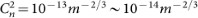. To estimate the turbulence effects on a laser beam, it is necessary to evaluate the Rytov variance, defined as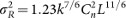where k is the modulus of the wave-vector and L the length of the path. Indicatively, one has strong or weak effects fororrespectively26. The optical beam is subjected to significant wandering and intensity speckles are observed at the receiver whenovertakes unity: the weaker is the level of turbulence, the longer has to be the link in order to apply our method.

For the link between La Palma and Tenerife we have estimated a night-time average structure constant: this value is consistent with the values obtained in other studies, i.e.27. Recently, in28 aoscillating between ≈ 5 · 10−16m−2/3 and ≈ 4 · 10−17m−2/3 has been reported. Although a detailed analysis of the turbulence strength would necessarily require from time to time a (hardly achievable) value of the structure constant for every part of the link, from these estimations one can safely draw the conclusion that due to the length of the channel we are working in the condition of large Rytov variance. By our estimation ofand using the 143 km length of the Canary Island link, we hadsuch that the condition for the speckle pattern formation was always satisfied.

Since the eddies are continuously moving according the unpredictable turbulent flow of the atmosphere, the distribution of the scintillation peaks in the receiver plane evolves randomly. So, for the purpose of random number generation, we acquired images with a CCD camera (Thorlabs DCC 1545 CMOS camera 1280 × 1024 pixels) at 12 and 25 frame per second (fps), with an exposure time of 3 ms, shorter than the characteristic time of fluctuations in order to not average out the dynamic of the process. A detailed analysis about the statistical independence of the frames and the stability of the link is presented in the Supplementary Information.

We now describe the method used to extract random numbers from the speckle positions: the CCD relevant pixels are labelled sequentially with an index s, s {1, …, N} and the nf speckle centroids of the frame f are elaborated (for details on the centroid extraction see Methods, subsection A). A threshold is set in order to skip those frames which could be affected by noise when the optical signal is too low, for example because an obstacle has crossed the path of the beam and then no light is detected. By considering then the pixels where a centroid fall in, an ordered sequencewith, can be formed. In this way the pixel grid can be regarded as the classical collection of urns - the pixel array - where the turbulence randomly throws in balls - the speckle centroids: a given frame f “freezes” one Sf out of the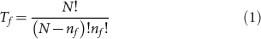possible and equally likely sequences of nf centroids. Among all of them, a given Sf can be univocally identified with its lexicographic index I(Sf)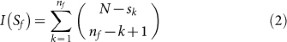with 0 ≤ I(Sf) ≤ Tf − 1. Basically, (2) enumerates all the possible arrangements which succeed a given centroids configuration and the TRNG distillates randomness by realizing the correspondence. Indeed, as an uniform RNG is supposed to yield numbers identically and independently distributed (i.i.d.) in a range [X, Y], as this method generates a random integer in the range [0, Tf − 1]. In order to obtain formula (2) we need to enumerate the combination of nf balls contained in N urns. The positions of the ball are identified with the integers. The number of possible combinations is. Let's first calculate the number of combinations that precede the given combination. This can be obtained by summing all the possible combinations in which the first ball falls in the positionswith, namely, plus all the combination in which the first ball is in s1 and the second ball is inwith, namely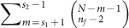, plus all the combination in which the first ball is in s1, the second in s2 and the third ball is inwithand so on. This number is given by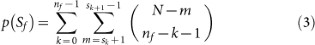where we defined s0 = 0. From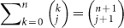, it can be shown that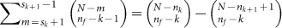so that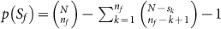. The number of combination that succeed Sf can be easily computed by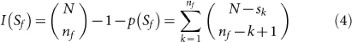where 0 ≤ I(Sf) < Tf. The number Tf − 1 represents then the upper bound to the uniform distribution of arrangement indexes which can be obtained by all the possible arrangements of nf centroids: the largest index, that is I(Sf) = Tf − 1, is obtained when all the centroids occupy the first urns of the grid.

To be conveniently handled, a binary representationof the random integers I(Sf) must be given. The simpler choice is to transform the integer I(Sf) in binary base, obtaining a sequence withbits. However, only if Tf mod 2i = 0 for i N, every frame f would theoretically provide stringsbits long. In general this is not the case and hence, all the frames withshould be accordingly discarded to avoid the so-called modulo bias. This issue, which clearly limits the rate of generation, can be solved by adopting the encoding function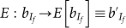developed by P. Elias29. With this approach, a string longer thanis mapped into a set of shorter sub-strings with equal probability of appearance. To convert the integer I(Sf), uniformly distributed in the interval [0, Tf − 1], into an unbiased sequence of bits, we may first consider the binary expansion of Tf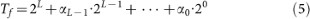whereand αk = 0, 1. Random bit strings are associated to I(Sf) according to the following rule: find the greatest m such that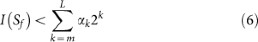and extract the first m bits of the binary expansion of I(Sf). By this rule, when I(Sf) < 2L, L bits can be extracted; when 2LI(Sf) < 2L + αL−12L−1, L − 1 bits can be extracted and so on; when I(Sf) = Tf − 1 and α0 = 1 (namely when m = 0) no string is assigned. It can be easily checked that this method, illustrated in Fig. 2, produces unbiased sequences of bits from integers uniformly distributed in the interval [0, Tf − 1].

This approach is optimal: the positions of nf centroids in N pixels can be seen as a biased sequence of N bits, with nf ones and Nnf zeros. The content of randomness of this biased sequence is h2(q) = −q log2q − (1 − q) log2(1 − q) with. By the Elias method it is possible to unbias the sequence in an optimal way: it can be shown that the efficiency, the ratio between the average length ofand N, reaches the binary entropy h2(q) in the limit of large N, limN→ ∞ η = h2(q). In this way it has been possible to preserve the i.i.d. hypothesis for the set [0, 1] maximizing the rate of the extraction.

The combinatorial approach here introduced allows a general approach compared to other techniques used to convert into random numbers the pixel coordinates of a detector. For example, in15, bi-dimensional random number arrays are obtained by converting in bits the position of those active pixels whose thresholds were adjusted in order to get the desired bivariate random distribution when illuminated with an uniform speckle pattern (i.e. to get an uniform distribution would be necessary to have half of the pixels over threshold and half below). With respect to the direct conversion approach, our method is more resilient, because by extracting the maximal entropy for a given frame, we do not need to constantly adjust the detector thresholds in function of the speckle pattern to get an uniform distribution of 0 s and 1 s.

### Analysis of the extracted bits

By implementing this technique with different configurations of masks and centroids, we were able to reach a maximum average rate of 17 kbit/frame (with a grid of 891000 urns and an average of 1600 centroids per frame). It is worth to stress that, for the present proof of principle, the distillation of random bits has been done off-line so, theoretically, having used a frame rate of 24 frame/s this method could provide a rate of 400 kbit/s using a similar camera and it could further increase by using a larger sensor.

The suitability of the method for random number generation depends on the statistical properties of the atmospheric turbulence over the time, in other words the stationarity and ergodicity of the physical process employed. It has been then fundamental to check the i.i.d. hypothesis for the numbers obtained by joining the bits belonging to frames of the same videos. A visual evidence that an overall uniformity is preserved during the whole acquisition time, it is given in Figure 3 where the distribution of 1.4 · 106 bytes obtained from a 671 frames video sample is plotted. If the bytes were used for cryptographic purposes, it is meaningful to consider the binary min-entropy hmin = maxi[−log2(pi)] where pi is the measured appearance probability of the byte i [0, 255]. A value ofbits per byte has been measured and this is compatible with the expected min-entropy for a sample of that size, that is Hmin = 7.946 ± 0.007. This experimental value is thus in agreement with the expected value from the theoretical prediction on uniform distribution, assessing an eavesdropper has no advantage with respect to random guessing (see Methods, subsection B, for a derivation of the expected min-entropy Hmin).

For assessing the randomness of a TRNG, in addition to a sound knowledge of the physical process employed, it is necessary to apply statistical tests in order to exclude the presence of defects caused by a faulty hardware. The theory and the positive results of the a selection of powerful tests are presented in the Methods and in Tables I and II. In particular, to obtain a confirmation of the i.i.d. hypothesis for the whole sets of bits, the numbers were thoroughly analyzed with three state-of-the-art batteries of tests whose results are reported in Table II. At present time, the TEST-U0133 is the most stringent and comprehensive suite of tests; among all, we chose a pair sub-batteries, Rabbit and Alphabit respectively, specifically designed to tests TRNGs. Note that, other batteries designed for algorithmic generators do not include tests sensitive to the typical TRNGs defects, such as correlations and bias. As it can be seen all the results were outside the limits ofor. The SP-800-2234 is developed by the NIST and it represents a common standard in RNG evaluation. For this suite, the files were partitioned in sub-strings 20 000 bits long: this length was chosen in order to obtain string sample sizes enough large such that with a significance level of α = 0.01, it is statistically likely to fail the tests in case of poor randomness (the sample sizes were then of 113, 207 and 559 strings respectively). Therefore, the tests suitable for this string size were applied with the NIST recommended parameters. Also in this case we registered successful results, being both the ratio between the sub-strings withand the total number of strings and the second-order test ondistribution, over the critical limits (passing ratios depends, time to time, on the number of strings analyzed, see Table II. For the goodness-of-fit test on the p-value distribution the limit is). Eventually, on the largest file obtained, we successfully applied also the AIS-3135 suite developed by the German BSI. The AIS-31 offers three sub-batteries of increasing difficulty which are intended to be applied on-line, that is to monitor the output of TRNG in order to detect failures and deviation from randomness: according to which level is passed, a TRNG can be considered preliminary suitable for different purposes (T0 pre-requisite level, T1 level for TRNGs used in connection with PRNG, T2 level for stand-alone TRNGs). From this analysis, where the more stringent and effective tests were applied and passed, the i.i.d. hypothesis resulted confirmed and strengthened.Table 2 Summary of the results of selected tests of batteries particularly effective in detecting defects in TRNG. The Alphabit and Rabbit batteries belong to the TESTU01: critical results are if or . The NIST SP-800-22 suite has passing ratio critical values for the three sets equal to 0.95575, 0.96618 and 0.97674 respectively. The test on the distribution of p-values must be . The AIS31 suite could be applied only on the larger set of bits: as it can be seen all the 263 tests of this suite were passed (N.P. correspond to those tests which are not possible to apply because of the files size, however those tests are already covered by the other batteries

## Discussion

As pointed out above, we are here addressing the two issues of introducing a method to extract good random numbers from random images and of generating these images from light propagating through the atmosphere. In particular, we exploited the propagation of the light over 143 km of turbulent atmosphere, giving rise to random speckle patterns at the receiver. The advantages of the method above presented in comparison with other TRNG resides in exploiting a good entropy source and in an efficient method to convert this entropy in a string of random bits. Indeed, when the conditions for strong optical turbulence are met, the scintillation images are resulting from a process that cannot be predicted, providing to a significant amount of entropy that may be extracted. In particular, the analytical models that are presently known to describe the dynamic of a turbulent fluid are not able to provide the evolution of the instantaneous intensity distribution. Moreover, if such models will be conceived, it is very presumable that they would require an extreme computational power to model the outcome of the propagation and still, according to the principle of the underlying nonlinear dynamics, maintaining the peculiar sensitivity on the initial conditions.

Other types of generators rely on small scale chaotic processes, such as sampling of laser intensity noise, but they must be carefully tuned in order to avoid the physical system to end in periodic trajectories and predictable outputs during the operation36. In particular, we can compare our method with the one proposed in30 and realized in31 where random numbers are obtained by sampling a detector illuminated with speckles produced by passing a laser beam between two rotating diffusers: such an approach however, as stressed by the authors themselves, could lead to periodicity due to the possibility that the same pattern repeats itself. Our TRNG is more resilient because we can safely exclude any periodicity of the speckle pattern.

A further advantage in exploiting optical beam propagation in turbulence is the fact that the physical process and the hardware are less prone to be influenced and controlled by an attacker, as is the case of generators which operate at the noise level limit. For example, generators based on measuring low amplitude voltage fluctuations in a resistor caused by the electronic thermal noise, can be easily influenced by modifying the environmental temperature37.

We now give two examples of application of our method. Our method could be directly applied in situations involving similar optical links, such as long range quantum communication experiments that require the generation of random numbers38,39. The second case is to apply the method by reducing the scale of the generator. The problem is then to individuate physical processes which can give rise to a speckle pattern randomly evolving in time. Different techniques, such as the dynamic light scattering, exploit speckle pattern analysis to infer a characterization of the diffusers, typically ranging from turbid media to organic tissues40,41. Such diffusers could be valid candidates for the purpose of continuous random number generation. By illuminating a colloidal suspension with a coherent light, random numbers could be extracted from the randomly evolving speckle pattern caused by the Brownian motions of the particles42.

Concerning our extraction technique, the algorithm here devised can be applied to any image from which it is possible to distill a spatial distribution of points. For example the lexicographic algorithm could be easily embedded in device which have a camera as mobile phones43,44 (cleary it would be necessary to investigate the possibility of finding a suitable kind of images to be taken with the phone camera from which i.i.d. random variables can be obtained). As last point we want to stress that the data obtained passed the most sensitive tests for TRNGs. The fact that here the randomness is generated without the need of any post-processing technique demonstrates the effectiveness of the present method.

## Methods

### Test of randomness

The output of a test on a bit string is another random variable with a given distribution of probability, the so-called test statistic. Hence, theare computed, namely the probability of getting an equal or worse test statistic, holding true the i.i.d. hypothesis. If theare smaller than some a priori defined critical value the tests are considered failed: these limits are usually chosen asand, corresponding to a confidence level of 99% and 99.9% respectively. Otherwise, whenever one obtainsequal or greater than these limits, the i.i.d. hypothesis for the tested string is assessed.

As first result of the statistical analysis, we present the outcomes of two tests, the frequency and the autocorrelation test respectively32. The first test checks whether the fraction of 0 s and 1 s departs from the expected value of 1/2 beyond the acceptable statistical limits. The second test evaluates whether the bit values depend on the neighboring bits. The output of both the tests (the serial autocorrelation with bit lag from 1 to 64) are test-statistics normally distributed and the analysis results are reported in Table I. From the frames we extracted and analysed 1483 strings 20 000 bits long (this string size has been selected for two main reasons: the first one in order to have a string sample large enough to comply the significance level both α = 0.01 (at least 100 elements) and α = 0.001 (at least 1 000). The second reason is because this string size is commonly used in standard tests suits such as FIPS-140-1 and AIS31, such that by passing or failing the above tests helps to understand the odds to pass also deeper statistical tests): the number of test statistics the i.i.d. hypothesis does not hold for (with a confidence of 99% and 99.9%, corresponding to ±2.58σ and ±3.29σ respectively) are inside the critical limits of statistical fluctuations, confirming the uniformity and the absence of correlations of the numbers. The main consequence of major defects at single bits level, is an even repartition of the Hamming weights which allows to pass also the so-called serial tests for the uniform distribution of many bits words. Applied on 2-bits, 2-bits overlapped and three 3-bits words the tests were all passed, as shown in Tab. I.

### Image processing

To extract the randomness from the frames of the videos, typical algorithms for image analysis which allows to compute several so-called digital moments were employed. More precisely, given E the number of bits used by the acquisition software to encode the intensity (color) levels of monochromatic light on the active area m·n of the sensor, we can consider the recorded image as a two variables function I(x, y) where x {0, …, m}, y {0, …, n} and I(x, y) {0, …, 2E}. The (j, k)th moment of an image is then defined as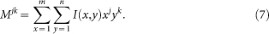The center of gravity C (also known as centroid) of an image is then located at position (,) where the coordinates are accordingly given by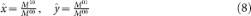We applied then a technique for instance used in Biology to count the number of cells in biological samples. Indeed in images composed by distinguishable components (as coloured cells on a uniform background), it is possible to locally calculate the centroids Ci of those components, by binarizing the intensity level, i.e. by setting E = 1 and then evaluating the moments on the closed subsets Si = {(x, y)|I(x, y) = 1}, that is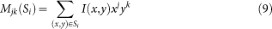where the index i runs on the different elements of the image.

To extract more randomness from the geometrical pool of entropy, the intensity profile of the frames has been partitioned into eight different sub-levels. We treated separately every different intensity level, L, as a source of spots; more specifically then we generated sets SL,i out of the L {1, …, 8} levels. For a given L and a spot i the coordinates of a centroids are then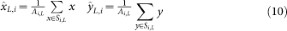where Ai,L simply the area of the spot, that is the total number of pixels which compose that spot. In order to remove edge effects due to the shape irregularities of the pupil, pixels close to irregular edges were removed.

### Min-entropy estimation

In this section we show how to estimate the expected min-entropy. In a sample with L bytes, the single byte occurrenceare random variables distributed according the Poisson distribution with mean. In order to estimate the expected min-entropy we need the distribution of the maximum of the occurrences and we can proceed as follow. Given a sample of n random variables X1, X2, …, Xn whose cumulative distribution function (CDF) is D(x) and the probability density function (PDF) is F(x), they can be re-ordered as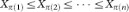: the Xπ(k) is called statistic of order k, such that min {X1, X2, …, Xn} = Xπ(1) and max {X1, X2, …, Xn} = Xπ(n). In order to derive the distribution function of an order k statistic, given h the number of Xix, one can note that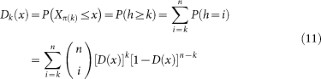Working with integer random variables the PDF is then obtained by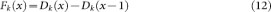Being interested in the byte frequencies maximal values, that is k = n, the previous equation becomes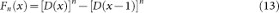In a sample with size L, the distribution of the maximum ℓM of the single byte occurrence ℓi can be computed by using the previous equation with,and n = 256: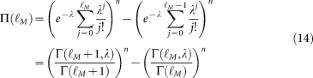The expected value and variance of the maximum of the ℓi's, are then easily evaluated by applying the definitionsandrespectively. With a sample size of L = 1399852 bytes and n = 256, the theoretical reference values are then evaluated to be 〈ℓM〉 = 5678.4 ± 29.4 counts with corresponding expected relative frequency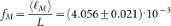. This value corresponds to a theoretical min-entropy of Hmin = −log2fM = 7.946 ± 0.007 bits per byte. If the obtained experimental min-entropy is compatible with the predicted theoretical value, the sample can be considered as uniformly distributed.

## References

1. Petrie, C. & Connelly, J. A noise-based IC random number generator for applications in cryptography. IEEE Trans. Circuits Syst. I Fundam. Theory Appl. 47, 615–621 (2000).

2. Reidler, I., Aviad, Y., Rosenbluh, M. & Kanter, I. Ultrahigh-speed random number generation based on a chaotic semiconductor laser. Phys. Rev. Lett. 103, 024102 (2009).

3. Sunar, B., Martin, W. & Stinson, D. A provably secure true random number generator with built-in tolerance to active attacks. IEEE Trans. Comput. 56, 109–119 (2007).

4. Stojanovski, T. & Kocarev, L. Chaos-based random number generators-part I: analysis. IEEE Trans. Circuits Syst. I Fundam. Theory Appl. 48, 281–288 (2001).

5. Fürst, M. et al. High speed optical quantum random number generation. Opt. Express 18, 13029–37 (2010).

6. Stipcevic, M. Quantum random number generators and their applications in cryptography. preprint [arXiv:1103.4381] (2011).

7. Vallone, G., Marangon, D. G., Tomasin, M. & Villoresi, P. Self-calibrating quantum random number generator based on the uncertainty principle. preprint [arXiv:1401.7917] (2014).

8. Fiorentino, M., Santori, C., Spillane, S., Beausoleil, R. & Munro, W. Secure self-calibrating quantum random-bit generator. Phys. Rev. A 75, 032334 (2007).

9. Pironio, S. et al. Random numbers certified by Bell's theorem. Nature 464, 1021–4 (2010).

10. Gabriel, C. et al. A generator for unique quantum random numbers based on vacuum states. Nat. Photonics 4, 711–715 (2010).

11. Schindler, W. [Evaluation criteria for physical random number generators]. Cryptographic Engineering, [25–54] (Springer, Berlin, 2009).

12. Dichtl, M. How to predict the output of a hardware random number generator. Paper presented at Cryptographic Hardware and Embedded Systems - CHES 2003, Cologne. Place of publication: Springer (2003, September).

13. Barthelemy, P., Bertolotti, J. & Wiersma, D. A Lévy flight for light. Nature 453, 495–8 (2008).

14. Goodman, J. W. Some fundamental properties of speckle. J. Opt. Soc. Am. 66, 1145 (1976).

15. Marron, J., Martino, A. J. & Morris, G. M. Generation of random arrays using clipped laser speckle. Appl. Opt. 25, 26 (1986).

16. Horstmeyer, R., Chen, R. Y., Judkewitz, B. & Yang, C. Markov speckle for efficient random bit generation. Opt. Express 20, 26394–410 (2012).

17. Pappu, R., Recht, B., Taylor, J. & Gershenfeld, N. Physical one-way functions. Science 297, 2026–30 (2002).

18. Ursin, R. et al. Entanglement-based quantum communication over 144 km. Nat. Phys. 3, 481–486 (2007).

19. Scheidl, T. et al. Violation of local realism with freedom of choice. Proc. Natl. Acad. Sci. 107, 19708–19713 (2010).

20. Ma, X.-S. et al. Quantum teleportation over 143 kilometres using active feed-forward. Nature 489, 269–273 (2012).

21. Capraro, I. et al. Impact of turbulence in long range quantum and classical communications. Phys. Rev. Lett. 109, 200502 (2012).

22. Kolmogorov, A. N. The local structure of turbulence in incompressible viscous fluid for very large Reynolds numbers. In Dokl. Akad. Nauk SSSR, 30, 299–303 (1941).

23. Fante, R. L. Electromagnetic beam propagation in turbulent media. In IEEE Proc. 63, 1669–1692 (1975).

24. Fante, R. L. Electromagnetic beam propagation in turbulent media-An update. In IEEE Proc. 68, 1424–1443 (1980).

25. Andrews, L. C. & Phillips, R. L. Laser Beam Propagation Through Random Media (SPIE Press, 2005).

26. Piazzolla, S. [Atmospheric Channel]. Near-Earth Laser Communications [237–270] (CRC Press, Boca Raton, 2009).

27. Comeron, A., Bara, J., Belmonte, A., Ruiz, D. & Czichy, R. Inter-islands optical link tests. IEEE Photonics Technol. Lett. 2, 380–381 (1990).

28. Schmitt-Manderbach, T. Long distance free-space quantum key distribution. Ph.D. thesis .(Ludwig Maximillian Universitat, Munich, 2007).

29. Elias, P. The efficient construction of an unbiased random sequence. Ann. Math. Stat. 43, 865–870 (1972).

30. Devos, F., Garda, P. & Chavel, P. Optical generation of random-number arrays for on-chip massively parallel Monte Carlo cellular processors. Opt. Lett. 12, 152–4 (1987).

31. Lalanne, P. et al. 2-D generation of random numbers by multimode fiber speckle for silicon arrays of processing elements. Opt. Commun. 76, 387–394 (1990).

32. Menezes, A. J., Van Oorschot, P. C. & Vanstone, S. A. Handbook of applied cryptography (CRC press, 2010).

33. Simard, R. & L'Ecuyer, P. Test U01: A Software Library in ANSI C for Empirical Testing of Random Number Generators. .ACM Transactions on Mathematical Software 33, article n. 22 (2007).

34. Rukhin, A., Soto, J., Nechvatal, J., Smid, M. & Barker, E. NIST Special Publication 800-22 Revision 1a: A statistical test suite for random and pseudorandom number generators for cryptographic applications. (2010), (Date of access: 01/03/2013) URL http://csrc.nist.gov/groups/ST/toolkit/rng/documents/SP800-22rev1a.pdf.

35. Killmann, W. & Schindler, W. A proposal for: Functionality classes for random number generators. (2011), (Date of access: 01/03/2013) URL https://www.bsi.bund.de/SharedDocs/Downloads/EN/BSI/Zertifierung/Interpretation/AIS31\_Functionality\_classes\_for\_random\_number\_generators.pdf\_\_blob=publicationFile.

36. Uchida, A. et al. Fast physical random bit generation with chaotic semiconductor lasers. Nat. Photonics 2, 728–732 (2008).

37. Soucarros, M., Canovas-Dumas, C., Clediere, J., Elbaz-Vincent, P., Real, D. Influence of the temperature on true random number generators. Paper presented at IEEE International Symposium on Hardware-Oriented Security and Trust (HOST). San Diego CA. Place of publication: IEEE (2011 June).

38. Schmitt-Manderbach, T. et al. Experimental Demonstration of Free-Space Decoy-State Quantum Key Distribution over 144 km. Phys. Rev. Lett. 98, 010504 (2007).

39. Yin, J. et al. Quantum teleportation and entanglement distribution over 100-kilometre free-space channels. Nature 488, 185–8 (2012).

40. Berne, B. J. & Pecora, R. Dynamic light scattering: with applications to chemistry, biology and physics (Dover Publications, London, 2000).

41. Rabal, H. J. & Braga Jr, R. A. Dynamic laser speckle and applications (CRC Press, Boca Raton, 2010).

42. Douglass, K. M., Sukhov, S. & Dogariu, A. Superdiffusion in optically controlled active media. Nat. Photonics 6, 834–837 (2012).

43. Krhovják, J., Matyas, V. & Svenda, P. The sources of randomness in mobile devices. Paper presented at Nordsec 2007: The 12th Nordic Conference on Secure IT Systems. Reykjavk. Place of publication: Reykjavik University, (2007 October).

44. Sanguinetti, B., Martin, A., Zbinden, H. & Gisin, N. Quantum random number generation on a mobile phone. preprint [arXiv:1405.0435] (2014).

## Acknowledgements

The authors wish to warmly thank for the help provided by Z. Sodnik of the European Space Agency and by C. Barbieri, S. Ortolani, D. Bacco and F. Gerlin of University of Padova as well as by the Instituto de Astrofisica de Canarias (IAC) and in particular F. Sanchez-Martinez, A. Alonso, C. Warden and J.-C. Perez Arencibia and by the Isaac Newton Group of Telescopes (ING) and in particular M. Balcells, C. Benn, J. Rey, A. Chopping and M. Abreu. This work has been carried out within the Strategic-Research-Project QUINTET of DEI-University of Padova and the Strategic-Research-Project QUANTUMFUTURE of the University of Padova.

## Author information

Authors

### Contributions

D.G.M., G.V. and P.V. realized the experimental part and devised the randomness extraction. D.G.M. performed the test on randomness versus the international standards. D.G.M., G.V. and P.V. contributed to the manuscript.

## Ethics declarations

### Competing interests

The authors declare no competing financial interests.

## Rights and permissions

Reprints and Permissions

• ### Exploiting Random Chemistry for Digital Security

• Davide G. Marangon

Matter (2020)

• ### Random number generation and distribution out of thin (or thick) air

• N Bornman
• , A Forbes
•  & A Kempf

Journal of Optics (2020)

• ### Practical True Random Number Generator Using CMOS Image Sensor Dark Noise

• Byung Kwon Park
• , Hojoong Park
• , Yong-Su Kim
• , Ju-Sung Kang
• , Yongjin Yeom
• , Changhui Ye
• , Sung Moon
•  & Sang-Wook Han

IEEE Access (2019)

• ### Real-Time Source-Independent Quantum Random-Number Generator with Squeezed States

• Thibault Michel
• , Jing Yan Haw
• , Davide G. Marangon
• , Oliver Thearle
• , Giuseppe Vallone
• , Paolo Villoresi
• , Ping Koy Lam